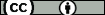## Quantitative estimates for positive linear operators in terms of the usual second modulus

Resumen: We give accurate estimates of the constants Cn(A(I),x) appearing in direct inequalities of the form |Lnf(x)-f(x)|=Cn(A(I),x)?2 f;s(x)/n, f?A(I), x?I, and n=1,2,., where Ln is a positive linear operator reproducing linear functions and acting on real functions f defined on the interval I, A(I) is a certain subset of such functions, ?2(f;·) is the usual second modulus of f, and s(x) is an appropriate weight function. We show that the size of the constants Cn(A(I),x) mainly depends on the degree of smoothness of the functions in the set A(I) and on the distance from the point x to the boundary of I. We give a closed form expression for the best constant when A(I) is a certain set of continuous piecewise linear functions. As illustrative examples, the Szàsz-Mirakyan operators and the Bernstein polynomials are discussed.
Idioma: Inglés
DOI: 10.1155/2015/915358
Año: 2015
Publicado en: Abstract and Applied Analysis 2015 (2015), 915358 [11 pp]
ISSN: 1085-3375

Factor impacto SCIMAGO: 0.55 - Applied Mathematics (Q3) - Analysis (Q3)

Financiación: info:eu-repo/grantAgreement/ES/MINECO-FEDER/MTM2011-23998
Tipo y forma: Article (Published version)You must give appropriate credit, provide a link to the license, and indicate if changes were made. You may do so in any reasonable manner, but not in any way that suggests the licensor endorses you or your use.

Este artículo se encuentra en las siguientes colecciones:
Articles